This file computes a clustering of the library branches based on principal components.

``````library(knitr)
setwd("/home/rburke/oboc/src/rcr-analysis/src/demographic")

### Project constants

``````# Load project constants
setwd("/home/rburke/oboc/src/rcr-analysis/src")
source("common.R")

library(caret)``````
``## Loading required package: lattice``
``## Loading required package: ggplot2``
``````library(stats)
library(gplots)``````
``````##
## Attaching package: 'gplots'``````
``````## The following object is masked from 'package:stats':
##
##     lowess``````
``````library(ggplot2)
library(GGally)``````
``````## Warning: replacing previous import by 'utils::capture.output' when loading
## 'GGally'``````
``## Warning: replacing previous import by 'utils::head' when loading 'GGally'``
``````## Warning: replacing previous import by 'utils::installed.packages' when
``## Warning: replacing previous import by 'utils::str' when loading 'GGally'``
``````library(RColorBrewer)
library(plyr)
library(cluster)
library(reshape2)``````

``````# Read the principal components of the demographic data
path.demo <- paste(RCR_DATA, "branch/branch-data-prcomp-",
DATA_VERSION, ".csv", sep="")

### Drop unused rows and columns

Remove non-demographic columns. Remove regional libraries as their demographics are just aggregations of the others. Create two data frames with 16 and 8 components, respectively.

``````# Drop the regional libraries and extraneous columns
code.drop <- c("H0", "S1", "W1")

demo.noH0 <- demo[demo\$Code!="H0",]
demo.noRG <- demo[!(demo\$Code %in% code.drop),]

branchPC16 <- demo.noRG[,c("PC1", "PC2", "PC3", "PC4", "PC5", "PC6", "PC7", "PC8",
"PC9", "PC10", "PC11", "PC12", "PC13", "PC14", "PC15", "PC16")]

branchPC8 <- demo.noRG[,c("PC1", "PC2", "PC3", "PC4", "PC5", "PC6", "PC7", "PC8")]``````

## Clustering computation

### A function to try clusterins

Using PAM - Partitioning Around Medoids.

``````# A function to explore different clustering

try_pam <- function(data,k) {
set.seed(327)
branch.pam <- pam(data, k)
diss <- daisy(data)
sil <- silhouette(branch.pam\$cluster, diss)
plot(sil)
return (branch.pam)
}``````

### k=3, 16 components

``````# k = 3
cl16_3 <- try_pam(branchPC16, 3)``````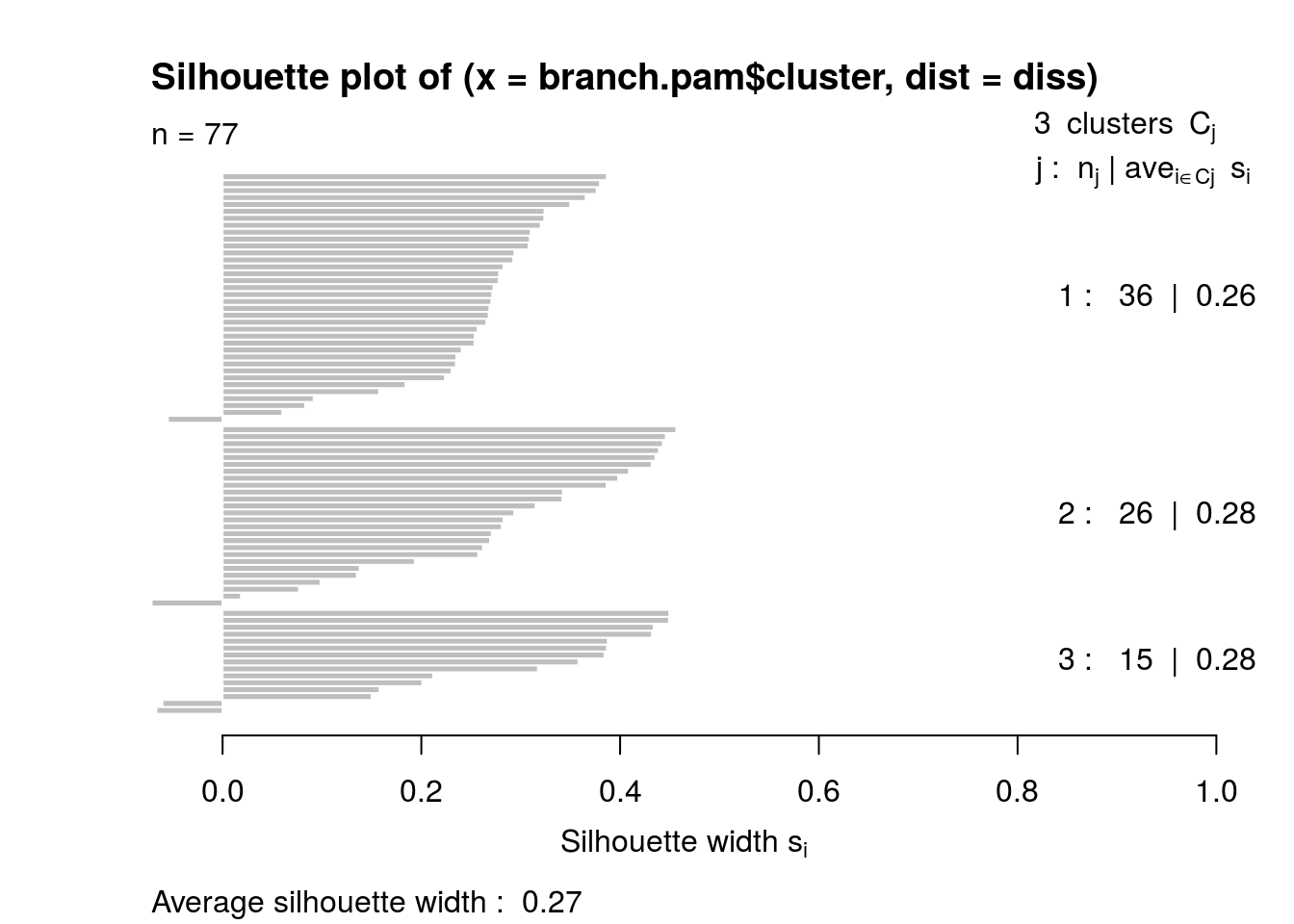### k=4

``````# k = 4
cl16_4 <- try_pam(branchPC16, 4)``````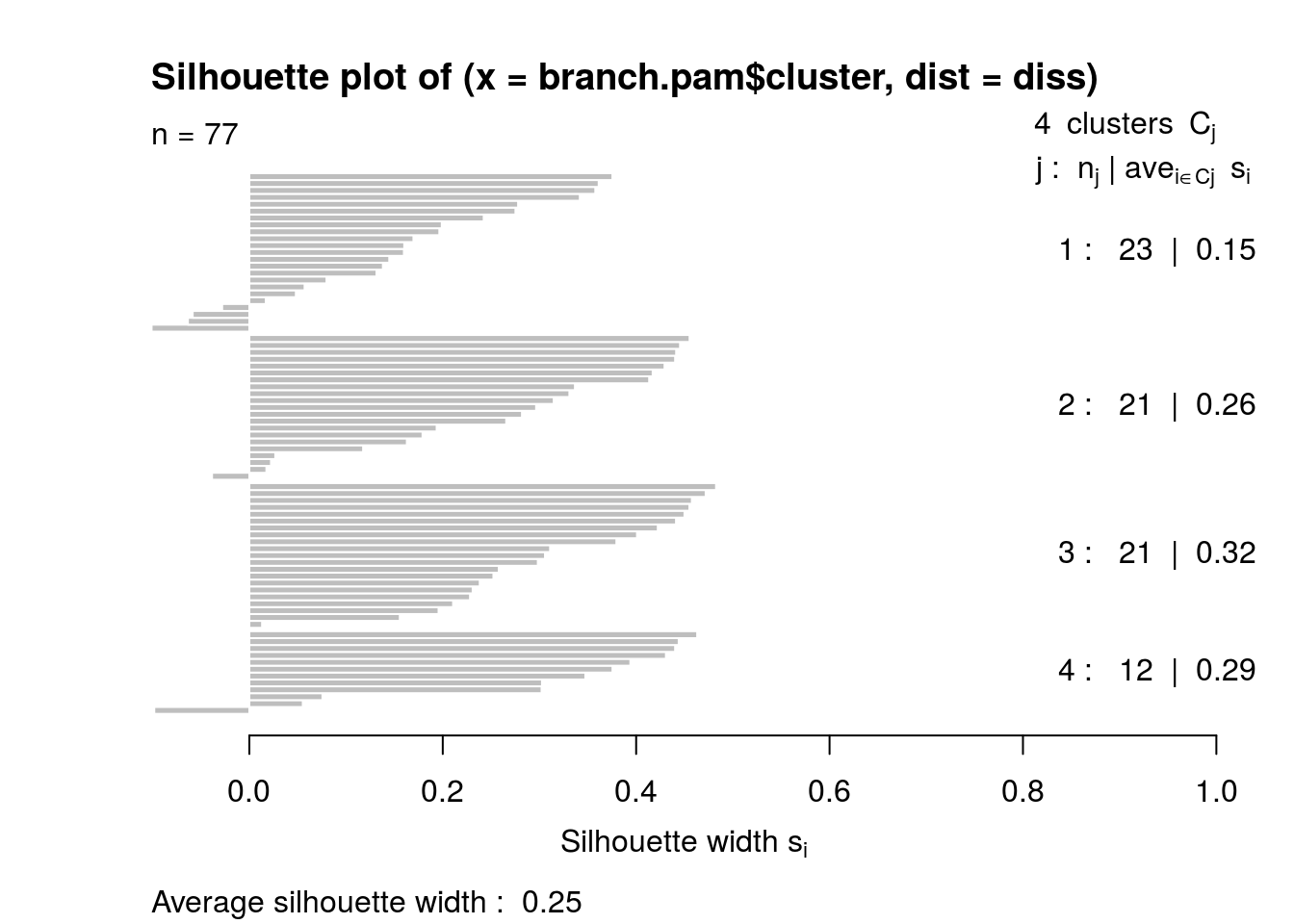### k=5

``````# k = 5
cl16_5 <- try_pam(branchPC16, 5)``````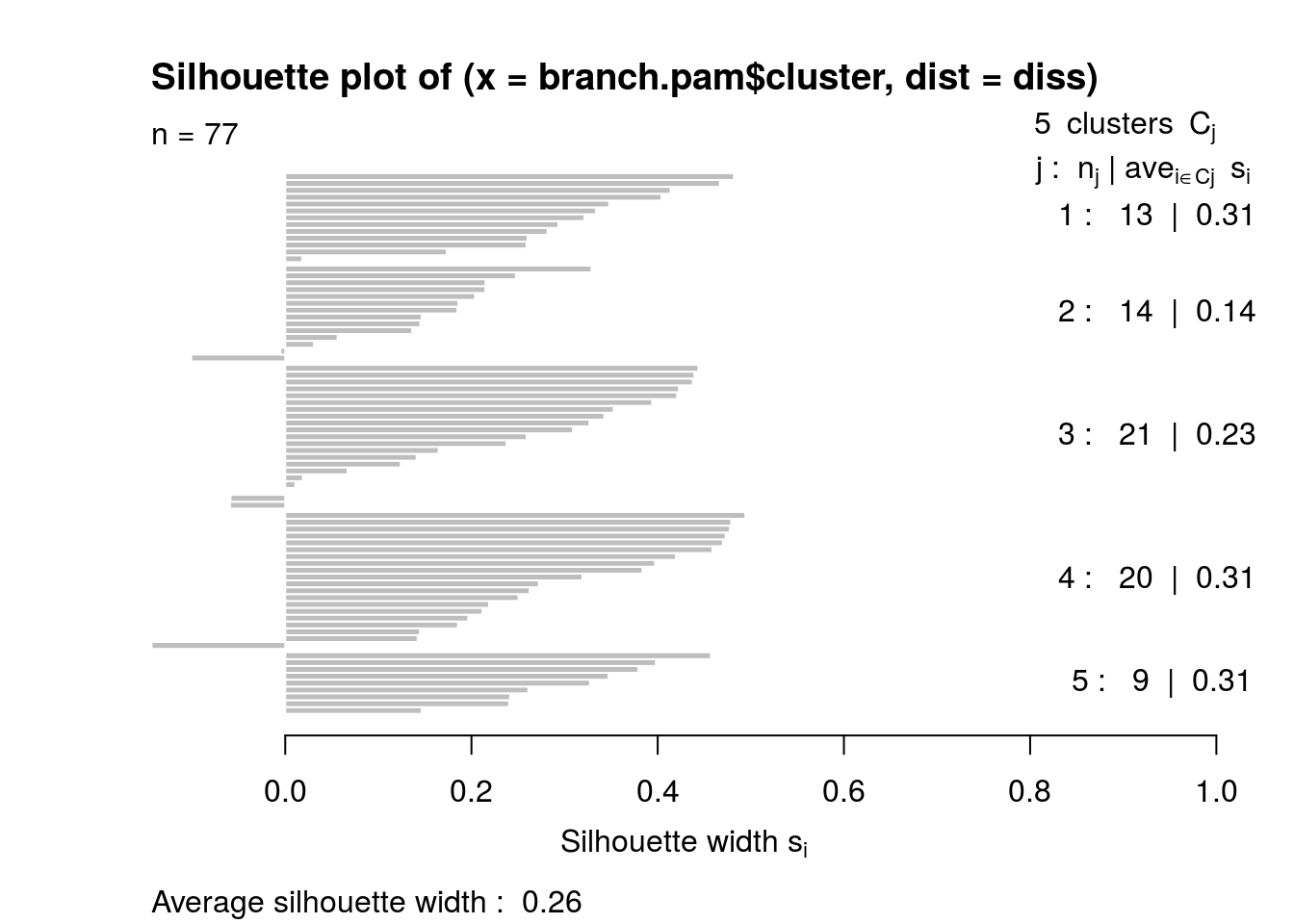### k=6

``````# k = 6
cl16_6 <- try_pam(branchPC16, 6)``````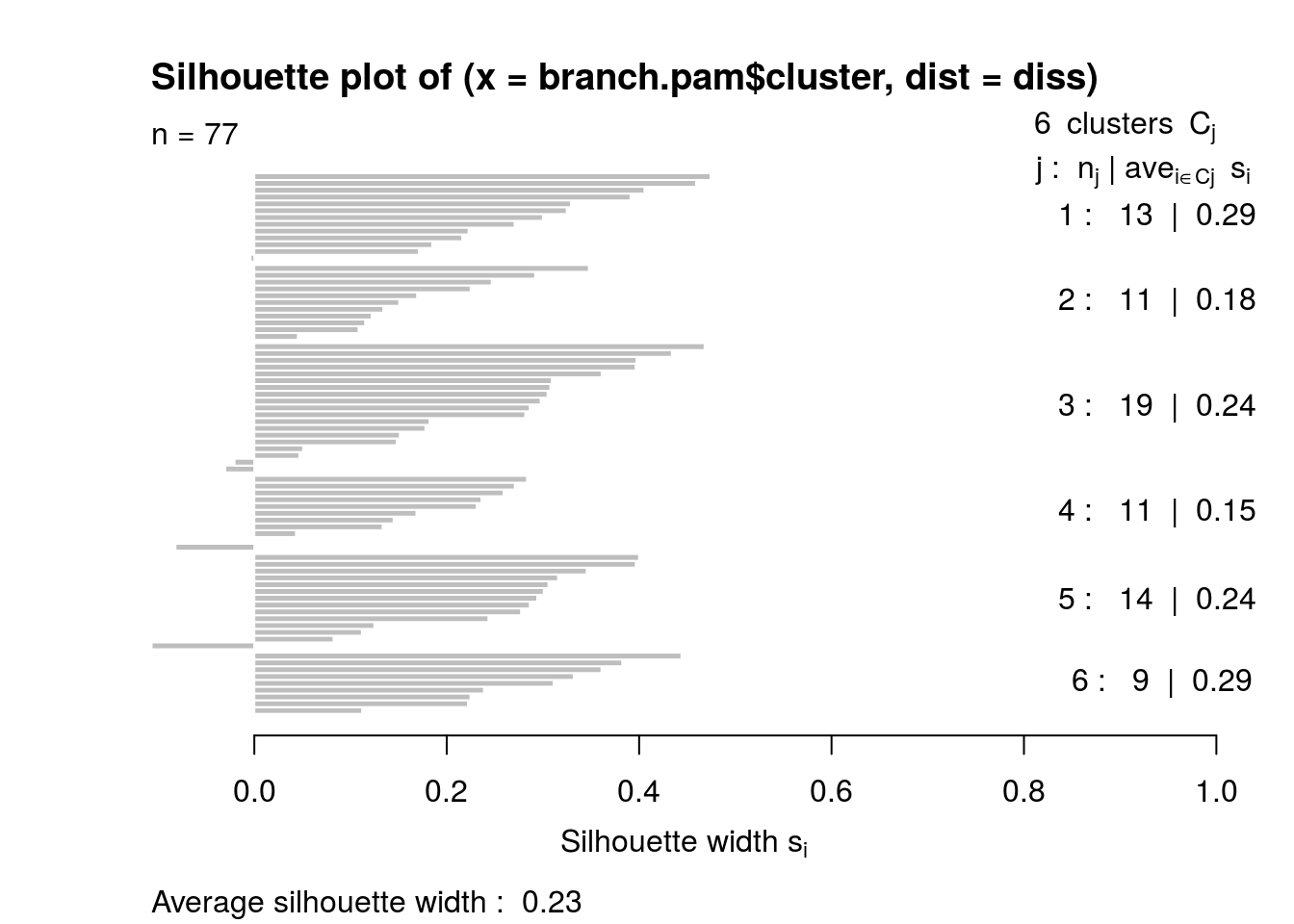### k=7

``````# k = 7
cl16_7 <- try_pam(branchPC16, 7)``````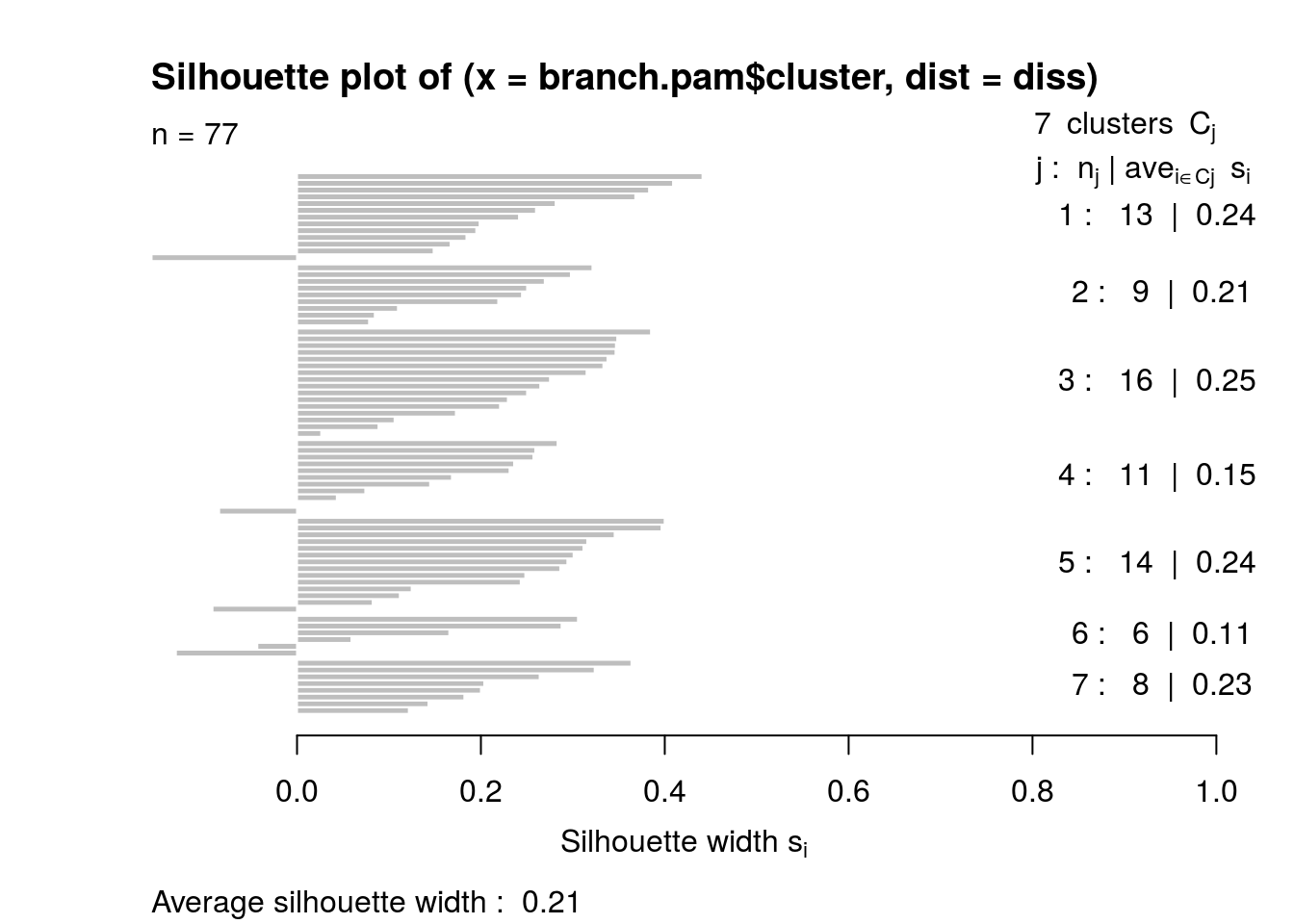The silhouette value peaks at k=5. Now trying with a smaller number of PCs. Perhaps less noise.

### k=4, 8 components

``````# k = 4
cl8_4 <- try_pam(branchPC8, 4)``````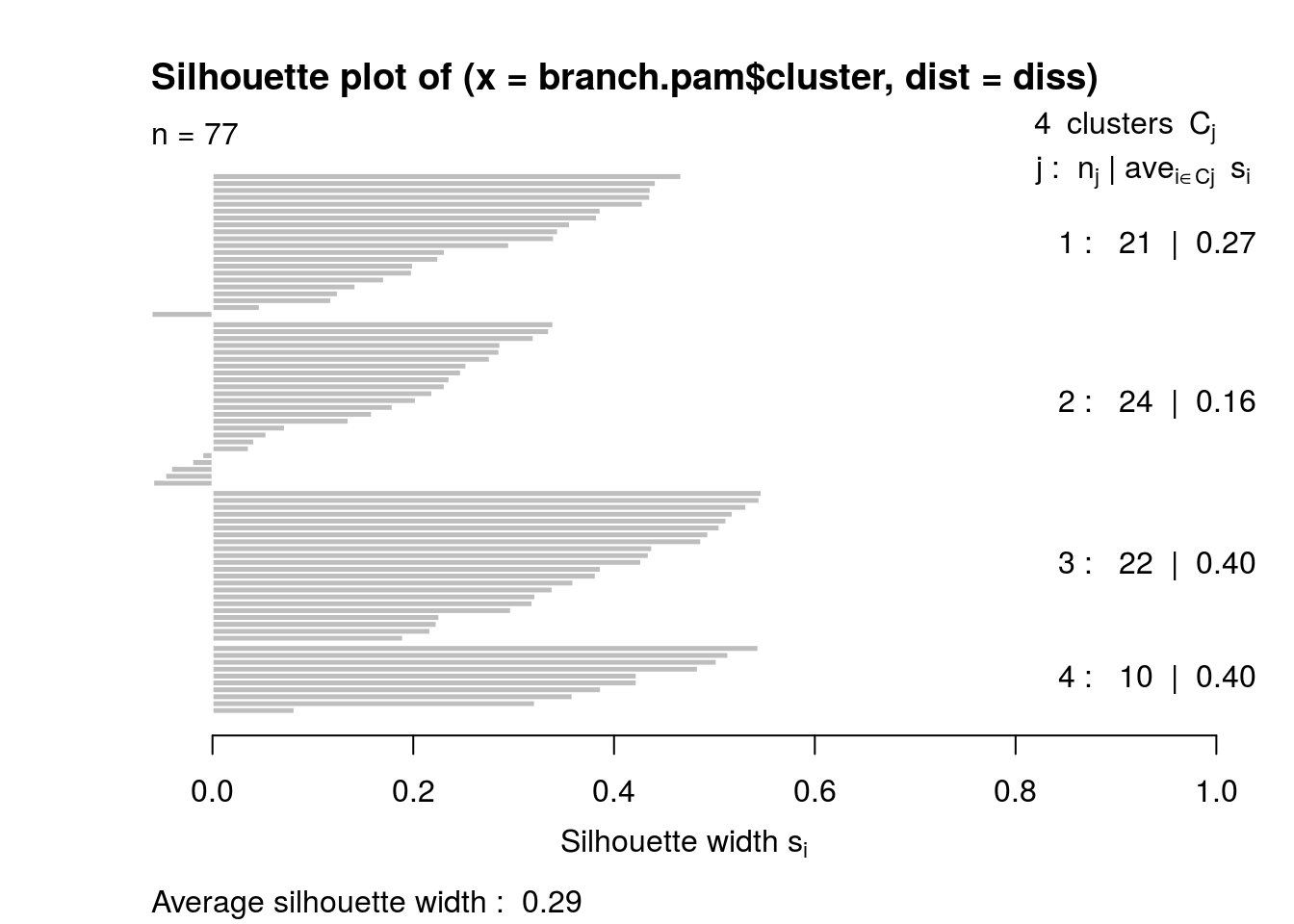### k=5

``````# k = 5
cl8_5 <- try_pam(branchPC8, 5)``````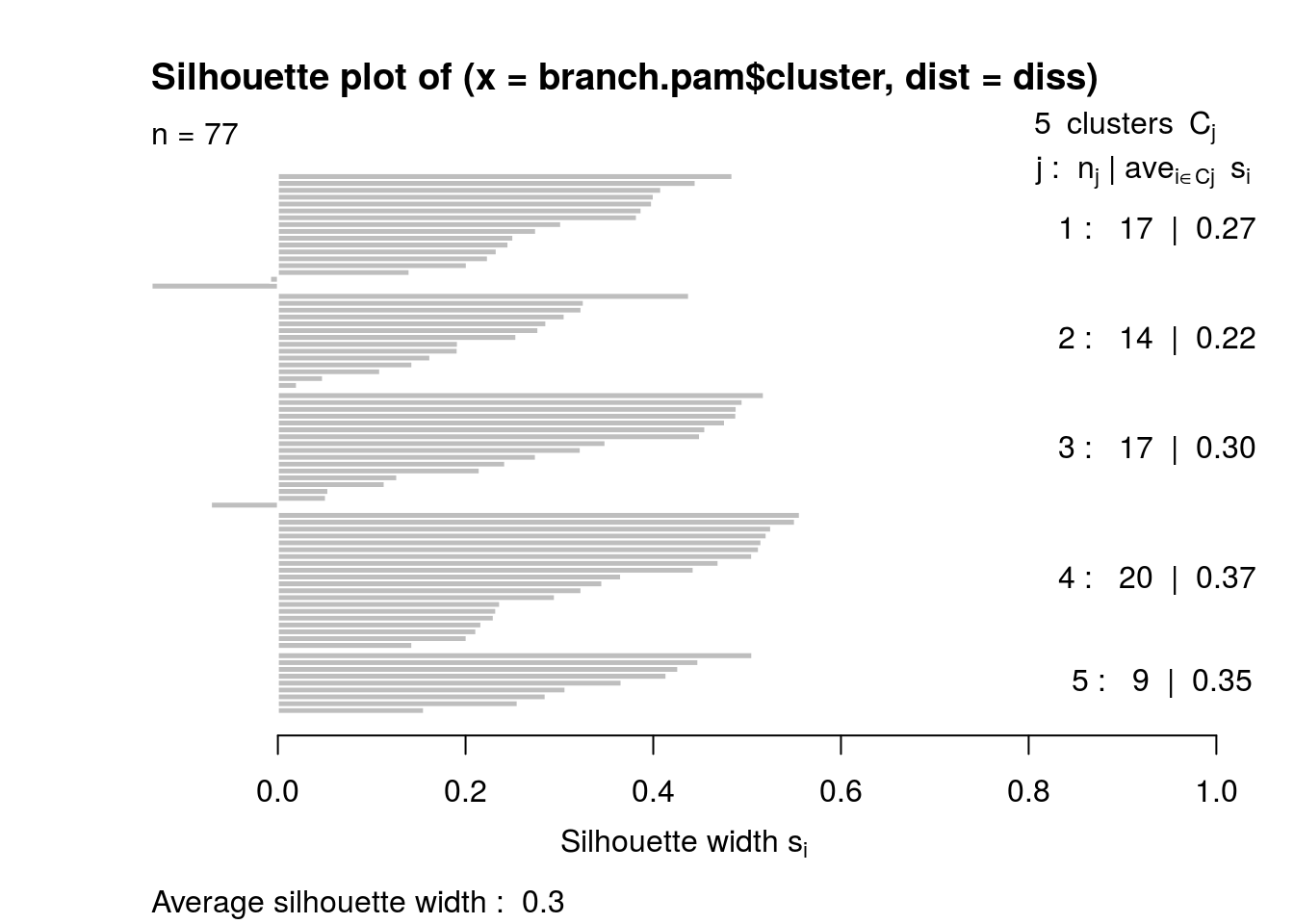### k=6

``````# k = 6
cl8_6 <- try_pam(branchPC8, 6)``````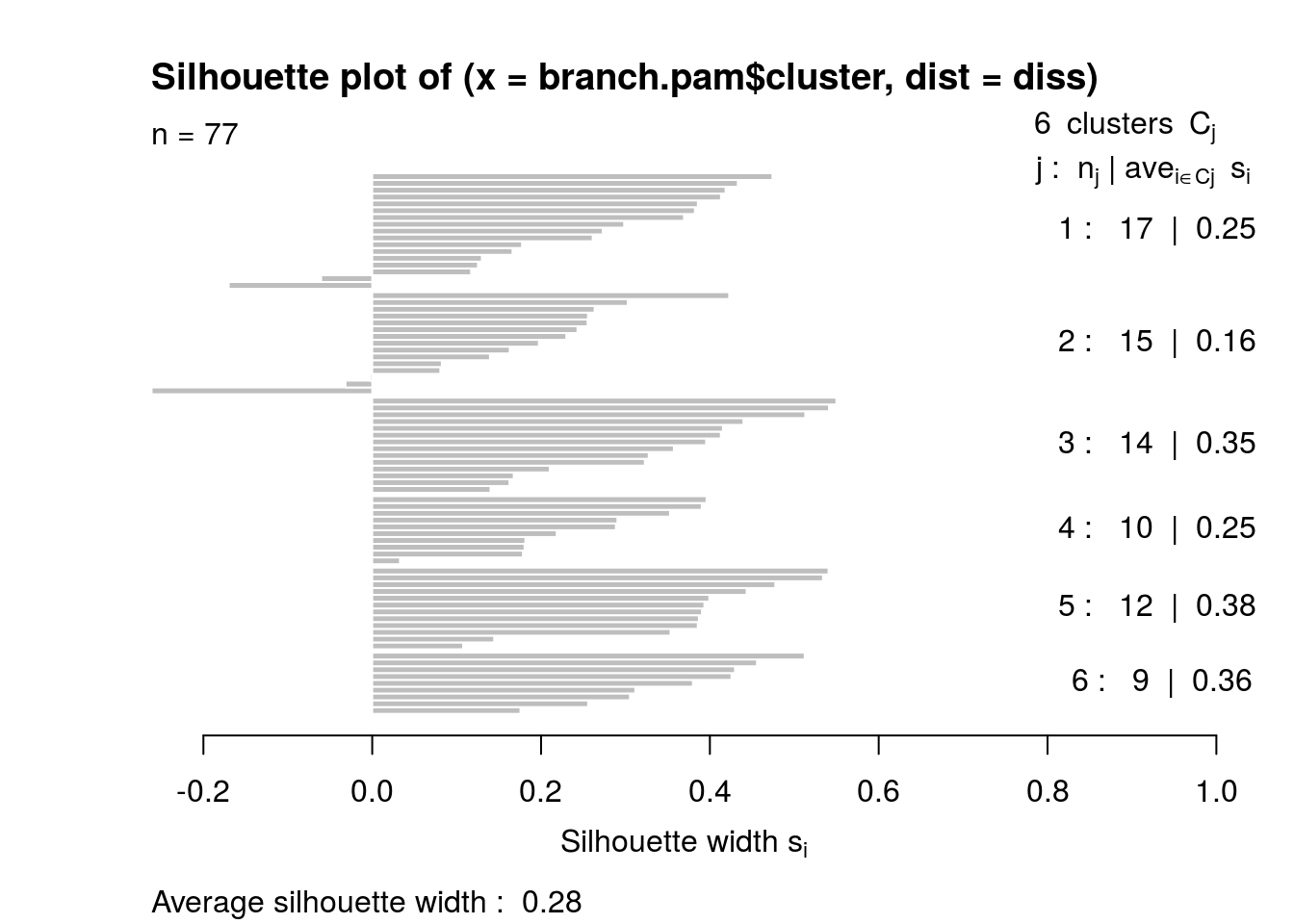### Choosing k = 5, with PC = 8

Adding back in the regional libraries as cluster 6.

``````# Slight improvement for PC8, k=5
branch.clust <- data.frame(Code=demo.noRG\$Code, Cluster=cl8_5\$clustering)
omitted <- data.frame(Code=code.drop, Cluster=6)
full.clust <- rbind(branch.clust, omitted)``````

### Saving

``````path.clust <- paste(RCR_DATA, "branch/branch-cluster-",
DATA_VERSION, ".csv", sep="")
write.csv(branch.clust, file=path.clust, row.names=FALSE)``````

### Examining the medoids

Note that the transform of PC2. This is the same transformation applied in the multi-level model. High PC^2 value reflects larger number of black and / or Latinx residents.

``````medoids <- cl8_5\$medoids

clust.info <- data.frame(Cluster=c("C1", "C2", "C3", "C4", "C5"), PC1=medoids[,1],
PC2X=medoids[,2], PC3=medoids[,3], PC4=medoids[,4])

clust.melt <- melt(clust.info, id.vars=c("Cluster"))
colnames(clust.melt) <- c("Cluster", "Component", "Weight")``````

### Plot of component weight in each cluster

``````p <- ggplot(data=clust.melt, aes(x=Cluster, y=Weight, fill=Component))
p <- p + geom_bar(stat="identity", position="dodge")
p <- p + scale_fill_discrete()
print (p)``````